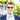# Object destructuring into class variables

March 27, 2022

## Solution

How to use object destructuring and directly assign the properties to TypeScript class variables.

``````class MyClass {
public name: string = '';
public age: number = 0;

constructor() {
// This is the important part :)
({userName: this.name, userAge: this.age} = this.getData());
}

private getData(): Data {
return {
userAge: 20,
}
}
}
interface Data {
userAge: number;
}``````

## Explanation

Let’s start in the beginning. Object destructuring allows you to extract properties from objects (and arrays) into new variables. The most basic example looks something like this

``````let {name, age} = { name: "Max", age: 21 };
console.log(name); // Max
console.log(age); // 21``````

The order does not matter for object properties, so this works just as well

``let {age, name} = { name: "Max", age: 21 };``

Sometimes you want to save the value into a variable with a different name. This can be done with a colon

``````let {name: nameVariable, age: ageVariable} = { name: "Max", age: 21 };
console.log(nameVariable); // Max
console.log(ageVariable); // 21``````

Before we can come back to our solution example with class properties, we need to look at one example before. It is possible to assign into variables that have been created earlier as well. However the following will result in an error

``````let nameVariable;
let ageVariable;

// This line will resolve in an error
{name: nameVariable, age: ageVariable} = { name: "Max", age: 21 };
console.log(nameVariable); // Max
console.log(ageVariable); // 21``````

What’s going on here? JavaScript treats `{...}` in a regular code flow as code blocks. This can be used to group statements. (But I don’t see a lot of devs actually doing this)

``````{
// code block start
let a = 12;
let b = 34;
console.log(a + b);
}``````

In order to tell JavaScript that our assignment is not a codeblock but an expression we can wrap the entire line with `(...)`

``````let nameVariable;
let ageVariable;

// This line will resolve in an error
({name: nameVariable, age: ageVariable} = { name: "Max", age: 21 });
console.log(nameVariable); // Max
console.log(ageVariable); // 21``````

And now we can use the last example in combination with TypeScript class variables.

``({userName: this.name, userAge: this.age} = this.getData());``Personal Blog written by Nicolas Gehlert, software developer from Freiburg im Breisgau. Developer & Papa. Github | Twitter